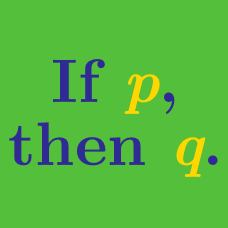Everyday Math

# Checking Cases

Which of the following could be an internal angle in a regular polygon?

$\begin{array}{r r l} &\text{I.}\ & 108 ^ \circ\\ & \text{II.}\ & 120 ^ \circ\\ &\text{III.} &167 ^ \circ\\ \end{array}$

(A)$\ \$ I only
(B)$\ \$ III only
(C)$\ \$ I and II only
(D)$\ \$ II and III only
(E)$\ \$ I, II, and III

If $n$ is a perfect square, which of the following could be true?

$\begin{array}{r r l} &\text{I.}\ & n\ \text{is a perfect cube.}\\ & \text{II.}\ & n^2 + 6\ \text{is a perfect square.}\\ & \text{III.}\ & n^2 + 1\ \text{is a multiple of 3.}\\ \end{array}$

(A)$\ \$ I only
(B)$\ \$ II only
(C)$\ \$ I and II only
(D)$\ \$ I and III only
(E)$\ \$ I, II, and III

If $x^3 < 8 x + 9$, which of the following could be a value of $x$?

$\begin{array}{r r l} & \text{I.} & 8\\ & \text{II.} & 0\\ & \text{III.} & -8\\ \end{array}$

(A)$\ \$ I only
(B)$\ \$ III only
(C)$\ \$ I and II only
(D)$\ \$ I and III only
(E)$\ \$ II and III only

For all numbers $a$ and $b$, let $a \heartsuit b$ be defined by $a \heartsuit b = ab + a -b$. For all numbers $x$ and $y$, which of the following must be true?

$\begin{array}{r r l} &\text{I.}\ & x \heartsuit y = y \heartsuit x\\ &\text{II.}\ & x \heartsuit y = (-y) \heartsuit (-x)\\ &\text{III.}\ & x \heartsuit y = (y+3) \heartsuit (x-3)\\ \end{array}$

(A)$\ \$ II only
(B)$\ \$ III only
(C)$\ \$ II and III only
(D)$\ \$ I, II, and III
(E)$\ \$ None of the statements

If $P$ is a prime number greater than 10, which of the following could represent another prime number for some value of $P$?

$\begin{array}{r r l} &\text{I.}\ &P+26\\ &\text{II.}\ & 5P\\ &\text{III.}\ & P^2 + 1\\ \end{array}$

(A)$\ \$ I only
(B)$\ \$ III only
(C)$\ \$ I and II only
(D)$\ \$ I and III only
(E)$\ \$ II and III only

×